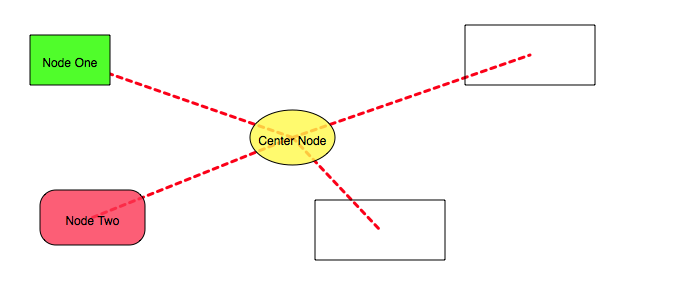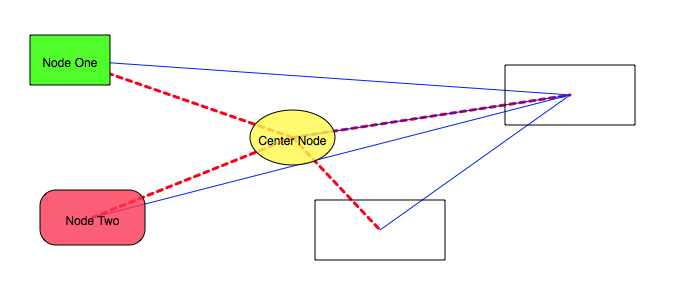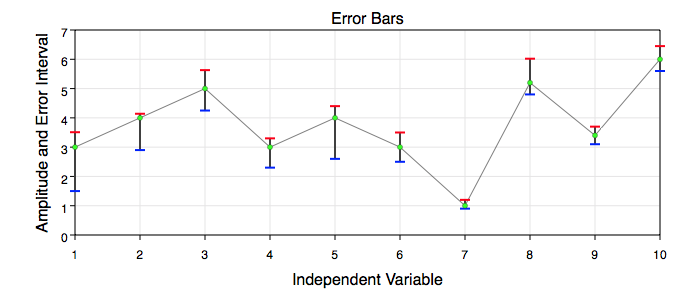Graph IDEComposites ► Network

A network is a connected sequence of graphics. When graphics are added to a Layer while a network is active then a network connection graphic is drawn between all graphics of that layer. Conceptually the idea is easy but learning how to turn on the network for the first time is not obvious. Also not obvious is the fact that error bars are a network type. The generalization of a network to error bars is very powerful, but that power comes at the cost of being required to understand the more abstract model of a network as defined in Graph IDE. Because of that, it might be useful to watch the following movie:

(Requires network connection www.vvidget.org)
For the full size movie click this link: Error Bars Movie.

Configuring a network is explained in the Inspector Editors ► Network section. The figure below shows a graph network where the yellow oval is a network hub.The following figure is the same as above except the upper-right rectangle is also a network hub with its own connection graphic which is a thin blue line. As you can see, introducing another hub produces a graph cycle.The figure below shows an error bar network where the center function graphic (curve) is connected to its upper and lower neighboring graphic's points.The easiest way to make an error bar network is to use the Error Bars assistant in the Chart Tasks and then to export to Graph IDE. Then you can dissect that output to discover how error bars work. Once you understand the nature of error bars then you can make simple or intricate error bar figures. Note that there can be multiple error bar hub nodes, the connection graphic can have caps, be vertical or horizontal or be an arbitrary graphic such as an image, oval or rectangle. There are many options and the Error Bars assistant instantiates only one.

The following table recaps some of the terminology and ideas of a network composite graphic.

 Term Description Connection A connection is a relationship between two nodes. The connection is displayed by a connection graphic and is synonymous with that graphic. Cycle When there are two or more hubs then the connection graphics can form a polygon which is called a cycle. Error Bars Error bars relate one graphic to another and hence are one type of connection. The error bars connect two or three nodes by those node's point values. Because error bars are point-oriented they only apply to Data Graphics. Graph A graph, in the context of a network, is a network and should not be confused with chart-type Graphs which are completely different. Sometimes a graph is also called an "object graph". Hub A hub is that node for which the connection graphic is defined. Each node can have its own connection graphic and hence any node can also be a hub although usually only one node is a hub. Neighbor A neighbor is a node that is next to another node. Unfortunately there are two meanings to the phrase "next to". For a graph, "next to" means all nodes in a layer. For error bars, "next to" means the nodes immediately before and after the sequence index of the node being considered. If the layer has nodes {n1 ... ni-1, ni, ni+1 ... nN} then the neighbors of ni are ni-1 and ni+1 for error bars but are the entire sequence of nodes for graphs. Network A network is a set of graphics on a layer that is related by a connection. In the context of a network the graphics are called nodes. Node A node is any graphic in the Layer. Satellite A satellite is any node that is not a hub for any given connection graphic. Subnetwork A subnetwork is a set of graphics that are connected where that set is a subset of a larger set of graphics. For example, a Group graphic connects only graphics within that group. A Layer does not connect to other layers so that subnetworks can be made to display by using different overlays. The Error Bars network type only connect to the two graphics before and after the hub graphic. In addition a node can be excluded from a network within a layer and the exclusion can then form a subnetwork.